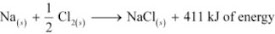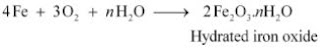# NCERT Solutions for Class 10 Science Chapter 1 Chemical Reactions and Equations

 Chapter Name NCERT Solutions for Class 10 Science Chapter 1 Chemical Reactions and Equations Topics Covered NCERT Intext QuestionsNCERT Exercise Solutions Related Study NCERT Solutions for Class 10 ScienceNCERT Revision Notes for Class 10 ScienceImportant Questions for Class 10 ScienceMCQ for Class 10 ScienceNCERT Exemplar Questions For Class 10 Science

### NCERT Exercises Solutions

1. Which of the statements about the reaction below are incorrect?
2PbO(s) + C(S) → 2Pb(s) + CO2(g)
(b) Carbon dioxide is getting oxidised.
(c) Carbon is getting oxidised.
(d) Lead oxide is getting reduced.
(i) (a) and (b)
(ii) (a) and (c)
(iii) (a), (b) and (c)
(iv) all

Solution

(i) (a) and (b)

2. Fe2O3 + 2Al → Al2O3 + 2Fe
The above reaction is an example of a
(a) combination reaction.
(b) double displacement reaction.
(c) decomposition reaction.
(d) displacement reaction.

Solution

(d) The given reaction is an example of a displacement reaction.

3. What happens when dilute hydrochloric acid is added to iron filings? Tick the correct answer.
(a) Hydrogen gas and iron chloride are produced.
(b) Chlorine gas and iron hydroxide are produced.
(c) No reaction takes place.
(d) Iron salt and water are produced.

Solution

(a) Hydrogen gas and iron chloride are produced. The reaction is as follows:
Fe(s) + 2HCl(aq) → FeCl2(aq) + H2

4. What is a balanced chemical equation ? Why should chemical equations be balanced ?

Solution

A reaction which has an equal number of atoms of all the elements on both sides of the chemical equation is called a balanced chemical equation. The law of conservation of mass states that mass can neither be created nor destroyed. Hence, in a chemical reaction, the total mass of reactants should be equal to the total mass of the products. It means that the total number of atoms of each element should be equal on both sides of a chemical equation. Hence, it is for this reason that chemical equations should be balanced.

5. Translate the following statements into chemical equations and then balance them.
(a) Hydrogen gas combines with nitrogen to form ammonia.
(b) Hydrogen sulphide gas burns in air to give water and sulphur dioxide.
(c) Barium chloride reacts with aluminium sulphate to give aluminium chloride and a precipitate of barium sulphate.
(d) Potassium metal reacts with water to give potassium hydroxide and hydrogen gas.

Solution

6. Balance the following chemical equations.
(a) HNO3 + Ca(OH)2 → Ca(NO3)2 + H2O
(b) NaOH + H2SO4 → Na2SO4 + H2O
(c) NaCl + AgNO3 →AgCl + NaNO3
(d) BaCl2 + H2SO4 → BaSO4 + HCl
Solution

7. Write the balanced chemical equations for the following reactions.
(a) Calcium hydroxide + Carbon dioxide → Calcium carbonate + Water
(b) Zinc + Silver nitrate → Zinc nitrate + Silver
(c) Aluminium + Copper chloride → Aluminium chloride + Copper
(d) Barium chloride + Potassium sulphate → Barium sulphate + Potassium chloride

8. Write the balanced chemical equation for the following and identify the type of reaction
in each case.
(a)Potassium bromide (aq) + Barium iodide (aq) → Potassium iodide (aq) + Barium bromide(s)
(b) Zinc carbonate (s) → Zinc oxide (s) + Carbon dioxide (g)
(c) Hydrogen (g) + Chlorine (g) → Hydrogen chloride (g)
(d) Magnesium (s) + Hydrochloric acid (aq) → Magnesium chloride (aq) + Hydrogen (g)
Solution

9. What does one mean by exothermic and endothermic reactions? Give examples.
Solution
Chemical reactions that release energy in the form of heat, light, or sound are called exothermic reactions.
Example: Mixture of sodium and chlorine to yield table saltIn other words, combination reactions are exothermic.
Reactions that absorb energy or require energy in order to proceed are called endothermic reactions.
For example: In the process of photosynthesis, plants use the energy from the sun to convert carbon dioxide and water to glucose and oxygen.

10. Why is respiration considered an exothermic reaction? Explain.
Solution
Energy is required to support life. Energy in our body is obtained from the food we eat. During digestion, large molecules of food are broken down into simpler substances such as glucose. Glucose combines with oxygen in the cells and provides energy. The special name of this combustion reaction is respiration. Since energy is released in the whole process, it is an exothermic process.

11. Why are decomposition reactions called the opposite of combination reactions? Write
equations for these reactions.
Solution
Decomposition reactions are those in which a compound breaks down to form two or more substances. These reactions require a source of energy to proceed. Thus, they are the exact opposite of combination reactions in which two or more substances combine to give a new substance with the release of energy.

12. Write one equation each for decomposition reactions where energy is supplied in the
form of heat, light or electricity.
Solution
(a) Thermal decomposition:

13. What is the difference between displacement and double displacement reactions? Write
equations for these reactions.
Solution
In a displacement reaction, a more reactive element replaces a less reactive element from a compound.
A + BX ⟶ AX + B; where A is more reactive than B
In a double displacement reaction, two atoms or a group of atoms switch place to from new compounds.
AB + CD ⟶ AD + CB
For example:
Displacement reaction :
CuSO4(aq) + Zn(s) → ZnSO4(aq) + Cu(s)
Double displacement reaction :
Na2SO4(aq) + BaCl2(aq) → BaSO4(s) + 2NaCl(aq)

14. In the refining of silver, the recovery of silver from silver nitrate solution involved displacement by copper metal. Write down the reaction involved.
Solution

15. What do you mean by a precipitation reaction? Explain by giving examples.
Solution
A reaction in which an insoluble solid (called precipitate) is formed is called a precipitation reaction.
Another example of precipitation reaction is :

16. Explain the following in terms of gain or loss of oxygen with two examples each.
(a) Oxidation
(b) Reduction
Solution
(a) Oxidation is the gain of oxygen.
For example :
In equation (i), H2 is oxidized to H2O and in equation (ii), Cu is oxidised to CuO.
(b) Reduction is the loss of oxygen.
For example :

17. A shiny brown - coloured element 'X' on heating in air becomes black in colour. Name the element 'X' and the black coloured compound formed.
Solution
'X' is copper (Cu) and the black - coloured compound formed is copper oxide (CuO). The equation of the reaction involved on heating copper is given below.

18. Why do we apply paint on iron articles?
Solution
Iron articles are painted because it prevents them from rusting. When painted, the contact of iron articles from moisture and air is cut off. Hence, rusting is prevented their presence is essential for rusting to take place.

19. Oil and fat containing food items are flushed with nitrogen. Why?
Solution
Nitrogen is an inert gas and does not easily react with these substances. On the other hand, oxygen reacts with food substances and makes them rancid. Thus, bags used in packing food items are flushed with nitrogen gas to remove oxygen inside the pack. When oxygen is not present inside the pack, rancidity of oil and fat containing food items is avoided.

20. Explain the following terms with one example each.
(a) Corrosion
(b) Rancidity
Solution
(a) Corrosion : Corrosion is defined as a process where materials, usually metals, deteriorate as a result of a chemical reaction with air, moisture, chemicals, etc. For example, iron, in the presence of moisture, reacts with oxygen to form hydrated iron oxide.This hydrated iron oxide is rust.
(b) Rancidity : The process of oxidation of fats and oils that can be easily noticed by the change in taste and smell is known as rancidity.
For example, the taste and smell of butter changes when kept for long. Rancidity can be avoided by :
1. Storing food in air tight containers
2. Storing food in refrigerators
4. Storing food in an environment of nitrogen

### NCERT Intext Questions

Page 6

1. Why should a magnesium ribbon be cleaned before burning in air ?
Solution
Magnesium is very reactive metal like (Na, Ca, etc.). When it expose to air it reacts with oxygen to form a layer magnesium oxide (MgO) on its surface.This layer of magnesium oxide is quite stable and prevents further reaction of magnesium with oxygen. The magnesium ribbon is cleaned by sand paper to remove this layer so that the underlying metal can be used for the reaction.

2. Write the balanced equation for the following chemical reactions.
(i) Hydrogen  + Chlorine → Hydrogen chloride
(ii) Barium chloride + Aluminium sulphate → Barium sulphate + Aluminium chloride
(iii) Sodium + water → Sodium hydroxide + Hydrogen
3. Write a balanced chemical equation with state symbols for the following reactions.
(i) Solutions of barium chloride and sodium sulphate in water react to give insoluble barium sulphate and the solution of sodium chloride.
(ii) Sodium hydroxide solution (in water) reacts with hydrochloric acid solution (in water) to produce sodium chloride solution and water.
Solution

Page 10

1. A solution  of a substance 'X' is used for white washing.
(i) Name the substance 'X' and write its formula.
(ii) Write the reaction of the substance 'X' named in (i) above with water.
Solution

2. Why is the amount of gas collected in one of the test tubes in Activity 1.7 double of the amount collected in the other ? Name this gas.
Solution
During the Electrolysis of water, hydrogen and oxygen is get separated by the electricity. Water (H2O) contains two parts hydrogen and one part oxygen. Since hydrogen goes to one test tube and oxygen goes to another, the amount of gas collected in one of the test tubes in double of the amount collected in the other.

Page 13

1. Why does the colour of copper sulphate solution change when an iron nail is dipped in it ?

Solution
When an iron nail is dipped in a copper sulphate solution, iron (which is more reactive than copper) displaces copper from copper sulphate solution forming iron sulphate, which is green in colour.
Therefore, the blue colour of copper sulphate solution fades and green colour appears.

2. Give an example of a double displacement reaction other than the one given in Activity 1.10.

Solution
Sodium carbonate reacts with calcium chloride to form calcium carbonate and sodium chloride.In this reaction, sodium carbonate and calcium chloride exchange ions to form two new compounds. Hence, it is a double displacement reaction.

3. Identify the substances that are oxidised and the substances that are reduced in the following reactions.
(i) 4Na(s) + O2(g)  → 2Na2O(s)
(ii) CuO(s) + H2(g) → Cu(s) + H2O(l)
Solution
(i) Sodium(Na) is oxidised as it gains oxygen and oxygen gets reduced.
(ii) Copper oxide (CuO) is reduced to copper (Cu) while hydrogen (H2)gets oxidised to water (H2O).